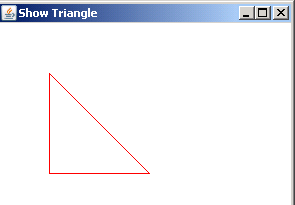Tutorials

# Draw a Triangle using a Line2D

To draw a triangle, we are using Line2D class of package java.awt.geom.*. This class provides a line segment in (x, y) coordinate space. We have draw three line segments using the class Line2D to create a triangle.

To draw a triangle, we are using Line2D class of package java.awt.geom.*. This class provides a line segment in (x, y) coordinate space. We have draw three line segments using the class Line2D to create a triangle.

# Draw a Triangle using a Line2D

This section illustrates you how to draw a triangle using a Line2D.

To draw a triangle, we are using Line2D class of package java.awt.geom.*. This class provides a line segment in (x, y) coordinate space. We have draw three line segments using the class Line2D to create a triangle.

The setPaint() method paints the line segment.

Here is the code of DrawTriangleUsingLine.java

 ``` import java.awt.*; import javax.swing.*; import java.awt.event.*; import java.awt.geom.Line2D; public class DrawTriangleUsingLine2D extends JApplet {   public void init() {   setBackground(Color.lightGray);   }   public void paint(Graphics g) {   Graphics2D g2d = (Graphics2D) g;   g2d.setPaint(Color.red);   g2d.draw(new Line2D.Double(50,150,150,150 ));   g2d.draw(new Line2D.Double(50,50,150,150 ));   g2d.draw(new Line2D.Double(50,50,50,150 ));   }   public static void main(String s[]) {   JFrame frame = new JFrame("Show Triangle");   JApplet applet = new DrawTriangleUsingLine2D();   frame.getContentPane().add("Center", applet);   applet.init();   frame.setSize(300, 250);   frame.show();   } }```

Output will be displayed as: